AP Calculus BC Review: Series Fundamentals

A series is what we call the sum of a sequence of numbers. Because most interesting sequences in calculus have infinitely many items, you can assume that most series will involve infinitely many terms as well. How in the world are you supposed to add up infinitely many terms, though???In this article, we’ll review the definition of a series. Then we’ll take a look at a few example problems from the AP Calculus BC exam. Once you have the series fundamentals down, then you’ll be able to tackle more advanced topics concerning series!

Series Fundamentals

Ok, let’s go over the basics.

Series and Sequences

First of all, the two terms, sequence and series, are not interchangeable.

A sequence is a list of numbers. Nothing more, nothing less! For all the essential details about sequences, you can check out: AP Calculus BC Review: Sequences and Convergence.

A series, on the other hand, is the sum of a sequence. You may see the sum written out term by term or in sigma notation. And both notations stand for the same thing.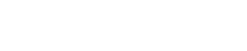We say that an is the general term for the series. Often, an will be expressed as a formula in terms of n.

Convergence and Divergence

If the series has a finite value, then we say it converges to that value. Otherwise, the series diverges.

A series may diverge in three different ways:

1. The sum gets larger and larger without bound as you add more terms. Then we say that the series diverges to infinity (∞).
2. The sum gets smaller and smaller (that is, large negative) without bound. In that case, we say that the series diverges to negative infinity (-∞)
3. The sum never stabilizes. As you add term after term, the value of the sum keeps jumping around or oscillating among multiple values. Here, we would say the series diverges (but not to ∞ nor -∞).

Partial Sums

The precise definition for convergence of a series has to do with its partial sums.

The kth partial sum for a series Σan is the sum of the first k terms of the series: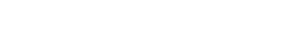So for example, the first four partial sums of a series are:Of course, the list of partial sums goes on forever. Next would be s5 having five terms, and so on.

By definition, the series Σan converges to a sum S if and only if the sequence of partial sums converges to S. That is,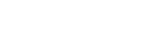A Few Good Examples

Here are some easy examples to get you started.

To Infinity (But Not Beyond…)

The series of all natural numbers (counting numbers) clearly diverges to infinity.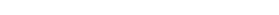That makes sense, right? If you keep adding larger numbers, the running total just gets bigger and bigger. More precisely, the partial sums are unbounded.In fact, any series whose general terms an do not tend to zero will diverge. In this case, the general term an = n itself blows up to ∞.

Geometry to the Rescue

On the other hand, the following series converges: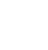This series is the sum of the reciprocals of the powers of 2.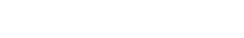In fact, this series converges to the value 2. It’s an example of a geometric series. There’s even a neat geometric argument to show why the sum is 2.The series 1 + 1/2 + 1/4 + 1/8 + …. converges to 2. Here, the area of the big rectangle (2 square units) is equal to the sum of areas of each square.

A Series That Can’t Make Up Its Mind

Finally, let’s take a look at a series that diverges due to the fact that the sum never stabilizes.Now, it’s tempting to say that the series converges to 0. But in reality, the sequence of partial sums oscillates between 1 and 0 forever.Because the partial sums never reach a definite limit, we say that this series diverges.

(By the way, this is another example in which the terms an do not approach zero as n → ∞. That fact alone automatically implies that the series diverges!)

Most of the time, it is very difficult to find a formula for the partial sums of a given series. However, in one important case, the partial sums seem to “collapse” like the sections of a telescope. In that case, it will be very easy to decide whether the series converges or diverges and to find its sum.Small telescopes used to be made to collapse for easy storage. The multiple sections of the telescope could slide into each other.

A telescoping series is one whose terms cancel with one another in a certain way.

For example, consider the following series.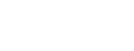Let’s write out the first four partial sums.I’ve highlighted the cancelling terms in red and blue. Once you cancel out those middle terms, there will only be two terms remaining in the partial sum, the first term 1/1 = 1, and the last term.

Let’s see how this plays out in general.Now we can actually find the sum series, based on the general formula for the partial sums!After all of the cancellations, this telescoping series collapses down to converge on the value 1.

Summary

Now that we’ve gone over the series fundamentals, let’s recap.

• A series is the sum of a sequence.
• Series can be expressed as a sum of (infinitely many) terms or by using sigma notation.• The kth partial sum of a series is the sum of its first k terms.
• A series converges to a sum S if and only if the sequence of its partial sums converges to S.

Improve your SAT or ACT score, guaranteed. Start your 1 Week Free Trial of Magoosh SAT Prep or your 1 Week Free Trial of Magoosh ACT Prep today!Author

•Shaun earned his Ph. D. in mathematics from The Ohio State University in 2008 (Go Bucks!!). He received his BA in Mathematics with a minor in computer science from Oberlin College in 2002. In addition, Shaun earned a B. Mus. from the Oberlin Conservatory in the same year, with a major in music composition. Shaun still loves music -- almost as much as math! -- and he (thinks he) can play piano, guitar, and bass. Shaun has taught and tutored students in mathematics for about a decade, and hopes his experience can help you to succeed!